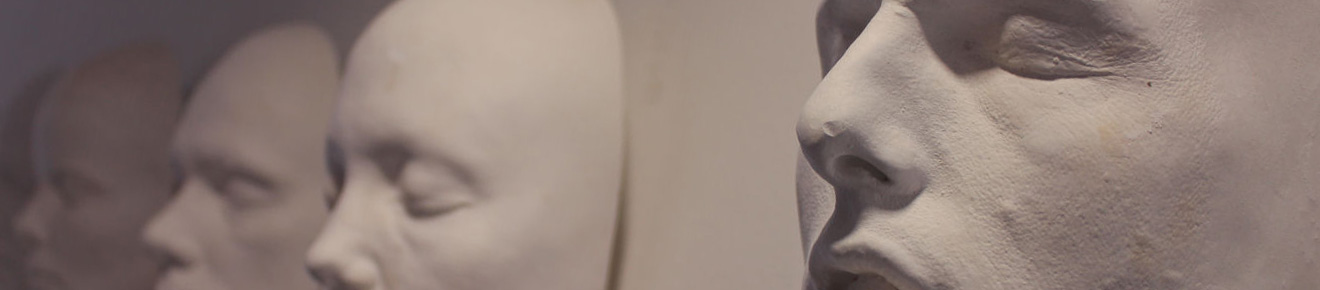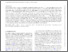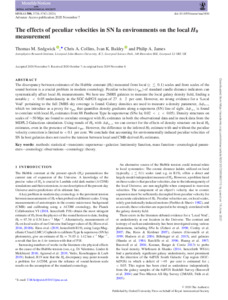# The effects of peculiar velocities in SN Ia environments on the local H0 measurement

Sedgwick, TM, Collins, CA, Baldry, IK and James, PA (2020) The effects of peculiar velocities in SN Ia environments on the local H0 measurement. Monthly Notices of the Royal Astronomical Society, 500 (3). pp. 3728-3742. ISSN 0035-8711Preview
Text
The effects of peculiar velocities in SN Ia environments on the local H0 measurement.pdf - Published Version

The discrepancy between estimates of the Hubble Constant ($H_{0}$) measured from local ($z \lesssim 0.1$) scales and from scales of the sound horizon is a crucial problem in modern cosmology. Peculiar velocities of standard candle distance indicators can systematically affect local $H_{0}$ measurements. We here use 2MRS galaxies to measure the local galaxy density field, finding a notable $z < 0.05$ under-density in the SGC-6dFGS region of $27 \pm 2$ %. However, no strong evidence for a 'Local Void' pertaining to the full 2MRS sky coverage is found. Galaxy densities are used to measure a density parameter, $\Delta \phi_{+-}$, which acts as a proxy for peculiar velocity ($v_{pec}$) by quantifying density gradients along a line-of-sight. $\Delta \phi_{+-}$ is found to correlate strongly with local $H_{0}$ estimates from Union 2.1 Type Ia SNe ($0.02 < z < 0.04$). Density structures on scales of $\sim 50$ Mpc are found to correlate most strongly with $H_{0}$ estimates in both the observational data and in mock data from the MDPL2-Galacticus simulation. Interpolating SN Ia $H_{0}$ estimates to their $\Delta \phi_{+-} = 0$ values, we can correct for the effects of density structure on the local $H_{0}$ estimates, even in the presence of biased peculiar velocities. For these particular observational data, we reveal a $< 0.1 \,\rm km\,s^{-1} Mpc^{-1}$ difference in the sample mean estimate compared to the value uncorrected for peculiar velocities. Our best estimate is then $74.9 \,\rm km\,s^{-1} Mpc^{-1}$. Using the mock data, the systematic uncertainty from these peculiar velocity corrections is estimated to be $0.3 \,\rm km\,s^{-1} Mpc^{-1}$. The dominant source of uncertainty in our estimate instead relates to Cepheid-based calibrations of distance moduli ($1.7 \,\rm km\,s^{-1} Mpc^{-1}$) and SN photometry ($0.7 \,\rm km\,s^{-1} Mpc^{-1}$).View Item